Latest Banking jobs   »   NIACL AO 2021 Result Out, Mains Exam Date For 300 AO Recruitment   »   Quantitative Aptitude Quiz For IBPS Clerk/NIACL...

# Quantitative Aptitude Quiz For IBPS Clerk/NIACL AO Prelims 2021- 9th September

Directions (1 – 5): Given below in each question there are two statements (I) and (II). You must determine; which statement is enough to give the answer of question. Also, there are five alternatives given, you have to choose one alternative as your answer of the questions:

Q1. What is area of the rectangle.
I. Length is 50% more than breadth.
II. Perimeter of square is 48 cm and breadth of rectangle is equal to side of square.
(a) only statement I
(b) Only statement II
(c) Both I and II together
(d) Either I or II alone
(e) Both statements together are not sufficient

Q2. What is age of Rahul after 2 years.
I. Average age of Arun and Neeraj is 24 years and ratio of age of Rahul to Arun is 2 : 3.
II. Neeraj is 4 years elder than Satish and ratio of age of Satish to Rahul is 1 : 2
(a) only statement I
(b) Only statement II
(c) Both I and II together
(d) Both statements together are not sufficient
(e) Either I or II alone

Q3. What is the speed of boat in still water when the upstream speed of boat is equal to the speed of stream?
I. Time required to cover certain distance upstream is 24 sec.
II. Time required to cover certain distance downstream is 8 sec.
(a) only statement I
(b) Only statement II
(c) Both I and II together
(d) Both together are not sufficient
(e) Either I or II alone

Q4. Find out the length of train X given that speed of train X is 20 m/sec.
I. Train X crosses another train Y moving in opposite direction in 6 sec and the speed of train Y is 50% more than the speed of train X.
II. Length of train Y is 50% less than length of train X.
(a) Both I and II together
(b) Only statement I
(c) Only statement II
(d) Both I and II together are not sufficient
(e) Either I or II alone

Q5. What is the total strength of company Adda247.
I. Ratio of male to female employees are 1 : 2
II. Total females are 280 and males are 50% of female.
(a) Both I and II together
(b) Only statement I
(c) Only statement II
(d) Both I and II together are not sufficient
(e) Either I or II alone

Directions (6-15): In the following questions two quantities are given for each question.
Compare the numeric value of both the quantities and answers accordingly.

Q6.Quantity I: x² – 6x + 8 = 0
Quantity II: y² + 2y – 8 = 0
(a) Quantity I > Quantity II
(b) Quantity I < Quantity II
(c) Quantity I ≥ Quantity II
(d) Quantity I ≤ Quantity II
(e) Quantity I = Quantity II or No relation

Q7. A man invested Rs. 8000 in scheme A for 4 years at Simple Interest which offers interest rate of 20% p.a and Rs. 7200 in scheme B for 3 years which offers 25% p.a at Simple interest.
Quantity I: Interest earned from scheme A
Quantity II Sum of interest earned from scheme ‘B’ and Rs. 640
(a) Quantity I > Quantity II
(b) Quantity I < Quantity II
(c) Quantity I ≥ Quantity II
(d) Quantity I ≤ Quantity II
(e) Quantity I = Quantity II or No relation

Q8. Sum of speed of boat in downstream and in upstream is 24 km/hr while speed of boat in still water is 200% more than speed of stream.
Quantity I: Time taken to cover 96km downstream
Quantity II: Time taken to cover 60km upstream
(a) Quantity I > Quantity II
(b) Quantity I ≤ Quantity II
(c) Quantity I ≥ Quantity II
(d) Quantity I = Quantity II or No relation
(e) Quantity I < Quantity II

Q9. Quantity I : Find the principal if the compound interest is charged on principal at the rate of 16⅔% per annum for two years and the sum becomes Rs 245.
Quantity II : A sum of Rs 500 amounts to Rs 620 in 4 yrs at simple interest. What will Rs 150 amount to if the rate of interest is same and time period is 2½ yrs.
(a) Quantity I > Quantity II
(b) Quantity II > Quantity I
(c) Quantity I >= Quantity II
(d) Quantity II >= Quantity I
(e) Quantity I = Quantity II or relation can’t be established

Q10.Quantity I: x² – 5x + 4 = 0
Quantity II: y² + 3y + 2 = 0
(a) Quantity I > Quantity II
(b) Quantity I < Quantity II
(c) Quantity I ≥ Quantity II
(d) Quantity I ≤ Quantity II
(e) Quantity I = Quantity II or No relation

Q11. (X – 4) men can complete a work in 5 day while (X + 5) men can complete the same work in 4 days. (Y – 15) women can complete a work in 6 days while (Y + 15) women can complete the same work in 3 days.
Quantity I: Value of ‘X’
Quantity II: Value of ‘Y’
(a) Quantity I > Quantity II
(b) Quantity I < Quantity II
(c) Quantity I ≥ Quantity II
(d) Quantity I ≤ Quantity II
(e) Quantity I = Quantity II or No relation

Q12. A and B starting a business together. B invested 60% more than that of A. Ratio of time period of investment of A and B is 8 : 9.
Quantity I: A’s share of profit out of total profit of Rs. 25,200.
Quantity II; B’s share of profit out of total profit of Rs. 14,000.
(a) Quantity I > Quantity II
(b) Quantity I < Quantity II
(c) Quantity I ≥ Quantity II
(d) Quantity I ≤ Quantity II
(e) Quantity I = Quantity II or No relation

Q13. Rs. ‘2X’ is invested half in scheme ‘A’ which offers 20% p.a. at S.I. while half in scheme ‘B’ which offer 10% p.a. at C.I. Total interest earned after 2 years is Rs. 4880.
Quantity I: Rs. (X + 2000)
Quantity II: 1.25X
(a) Quantity I > Quantity II
(b) Quantity I < Quantity II
(c) Quantity I ≥ Quantity II
(d) Quantity I ≤ Quantity II
(e) Quantity I = Quantity II or No relation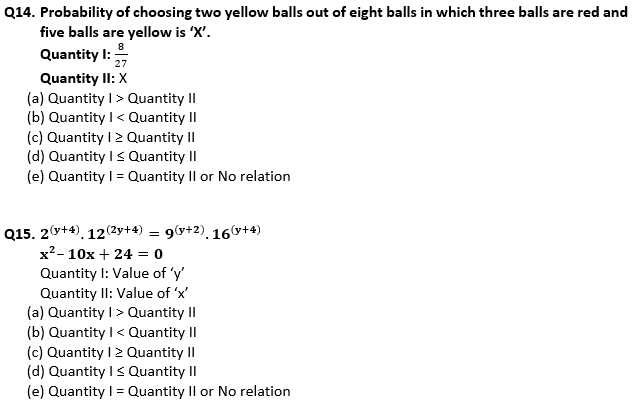Solutions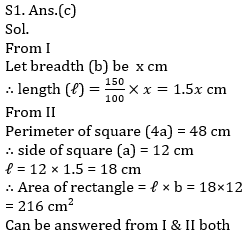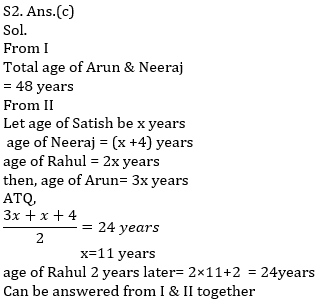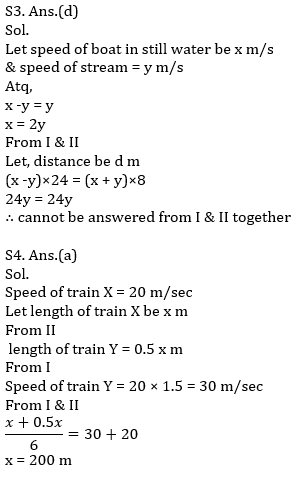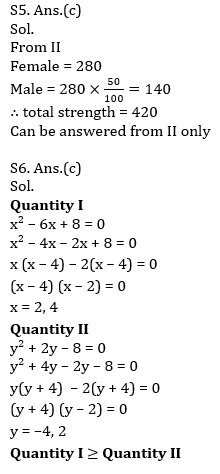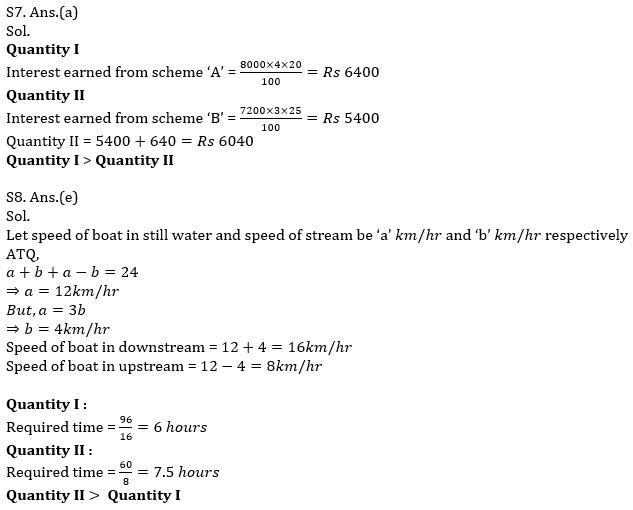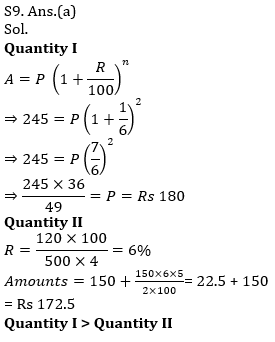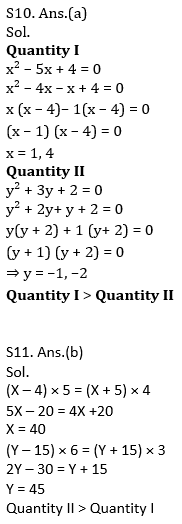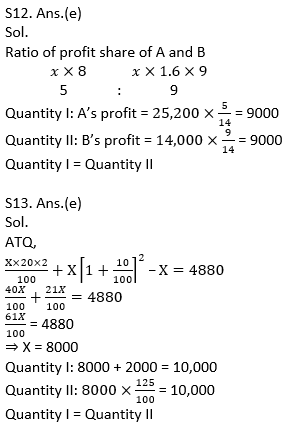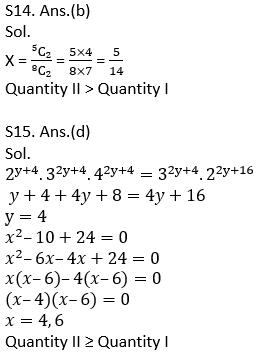Click Here to Register for Bank Exams 2021 Preparation Material#### Congratulations!Download Hindu Review of October 2021: Free PDF